" 他气的在出租车里走来走去 "

#  AMLP-Evaluation# Model evaluation and selection

## Dummy Classifier

Dummy Classifier is a classifier that makes predictions using simple rules.

This classifier is useful as a simple baseline to compare with other (real) classifiers. Do not use it for real problems.1

Dummy classifiers completely ignore the input data

• Dummy classifiers serve as a sanity check on your classifier's performance
• Provide a null metric baseline(e.g. null accuracy) which means it can be used to compare.
• Dummy classifiers should not be used for real problems.
from sklearn.dummy import DummyClassifier

# Negative class (0) is most frequent
dummy_majority = DummyClassifier(strategy = 'most_frequent').fit(X_train, y_train)
# Therefore the dummy 'most_frequent' classifier always predicts class 0
y_dummy_predictions = dummy_majority.predict(X_test)

y_dummy_predictions


most_frequent: predicts the most prequent label in the training set

stratified: random predictions based on training set class distribution

uniform: generates predictions uniformly at random

constant: always predicts a constant label provided by the user

dummy_majority.score


## Dummy regressors

strategy parameter options:

mean: predicts the mean of the training targets.

median: predicts the median of the training targets.

quantile: predicts a user-provided quantile of the training targets.

constant: predicts a constant user-provided value.

## Binary Prediction Outcomes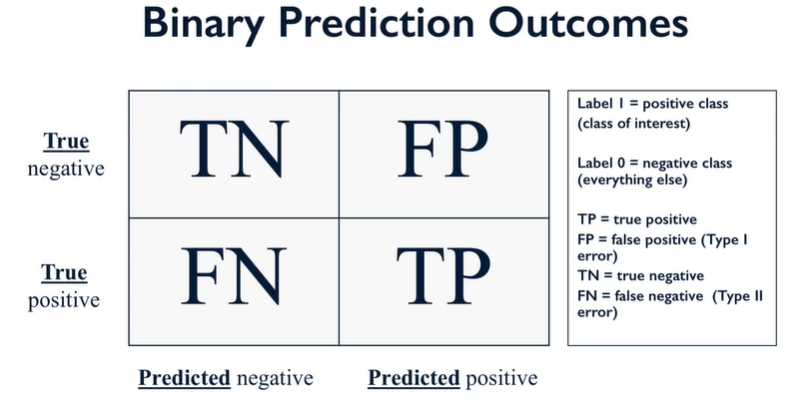Recall:

• True Positive Rate(TPR)
• Also known as Sensitivity
• Probability of detection

Specificity:

• Also known as False Positive Rate(FPR)

## Confusion Matrix for binary prediction

from sklearn.metrics import confusion_matrix

# Negative class (0) is most frequent
dummy_majority = DummyClassifier(strategy = 'most_frequent').fit(X_train, y_train)
y_majority_predicted = dummy_majority.predict(X_test)
confusion = confusion_matrix(y_test, y_majority_predicted)

print('Most frequent class (dummy classifier)\n', confusion)


Most frequent class (dummy classifier)
[[407 0]
[ 43 0]]

 # produces random predictions w/ same class proportion as training set
dummy_classprop = DummyClassifier(strategy='stratified').fit(X_train, y_train)
y_classprop_predicted = dummy_classprop.predict(X_test)
confusion = confusion_matrix(y_test, y_classprop_predicted)

print('Random class-proportional prediction (dummy classifier)\n', confusion)


Random class-proportional prediction (dummy classifier)
[[370 37]
[ 42 1]]

 svm = SVC(kernel='linear', C=1).fit(X_train, y_train)
svm_predicted = svm.predict(X_test)
confusion = confusion_matrix(y_test, svm_predicted)
print('Support vector machine classifier (linear kernel, C=1)\n', confusion)


Support vector machine classifier (linear kernel, C=1)
[[402 5]
[ 5 38]]

 from sklearn.linear_model import LogisticRegression

lr = LogisticRegression().fit(X_train, y_train)
lr_predicted = lr.predict(X_test)
confusion = confusion_matrix(y_test, lr_predicted)

print('Logistic regression classifier (default settings)\n', confusion)


Logistic regression classifier (default settings)
[[401 6]
[ 6 37]]

 from sklearn.tree import DecisionTreeClassifier

dt = DecisionTreeClassifier(max_depth=2).fit(X_train, y_train)
tree_predicted = dt.predict(X_test)
confusion = confusion_matrix(y_test, tree_predicted)

print('Decision tree classifier (max_depth = 2)\n', confusion)


Decision tree classifier (max_depth = 2)
[[400 7]
[ 17 26]]

• Search and information extraction in legal discovery
• Tumor detection
• Often paired with a human expert to filter out false positives

Precision-oriented

• Search engine ranking, query suggestion
• Document classification
• Many customer-facing tasks(users remember failures)
from sklearn.metrics import accuracy_score, precision_score, recall_score, f1_score
# Accuracy = TP + TN / (TP + TN + FP + FN)
# Precision = TP / (TP + FP)
# Recall = TP / (TP + FN)  Also known as sensitivity, or True Positive Rate
# F1 = 2 * Precision * Recall / (Precision + Recall)
print('Accuracy: {:.2f}'.format(accuracy_score(y_test, tree_predicted)))
print('Precision: {:.2f}'.format(precision_score(y_test, tree_predicted)))
print('Recall: {:.2f}'.format(recall_score(y_test, tree_predicted)))
print('F1: {:.2f}'.format(f1_score(y_test, tree_predicted)))


Accuracy: 0.95
Precision: 0.79
Recall: 0.60
F1: 0.68

# Combined report with all above metrics
from sklearn.metrics import classification_report

print(classification_report(y_test, tree_predicted, target_names=['not 1', '1']))


# Decision Function

## Varying the Decision Threshold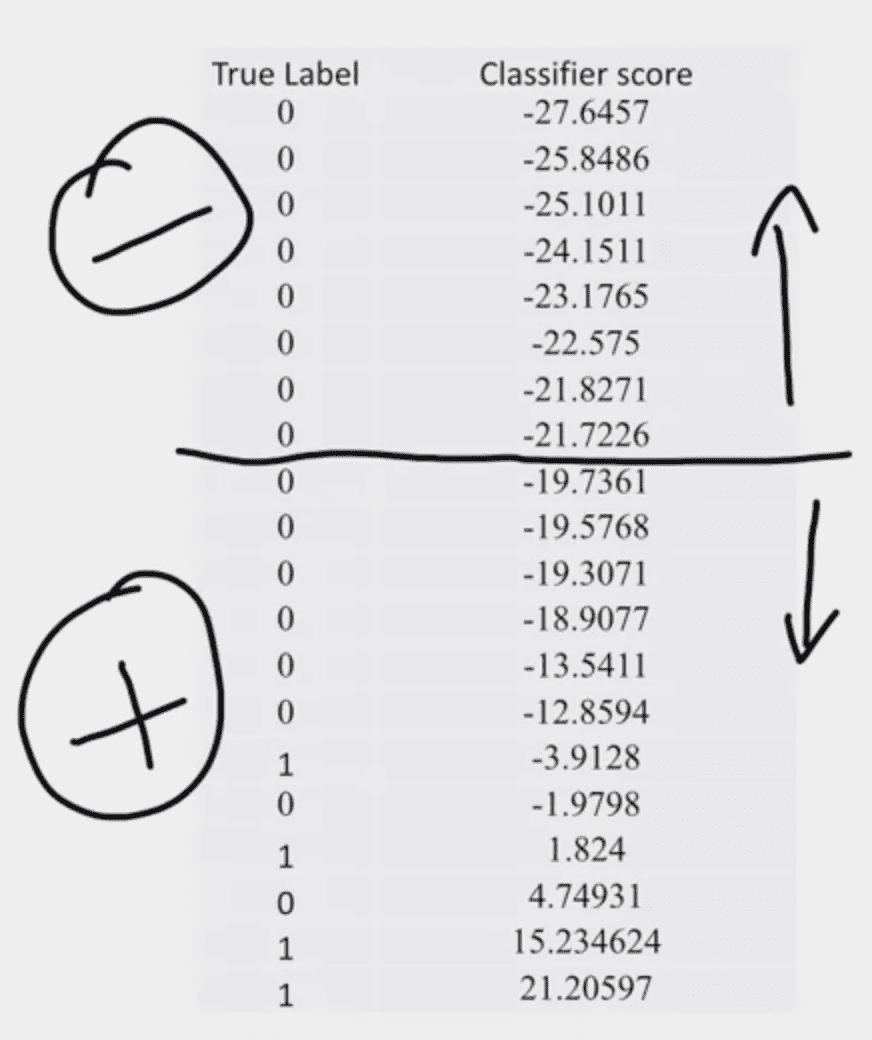vary the different threshold and evaluate the performance of models using precision and recall: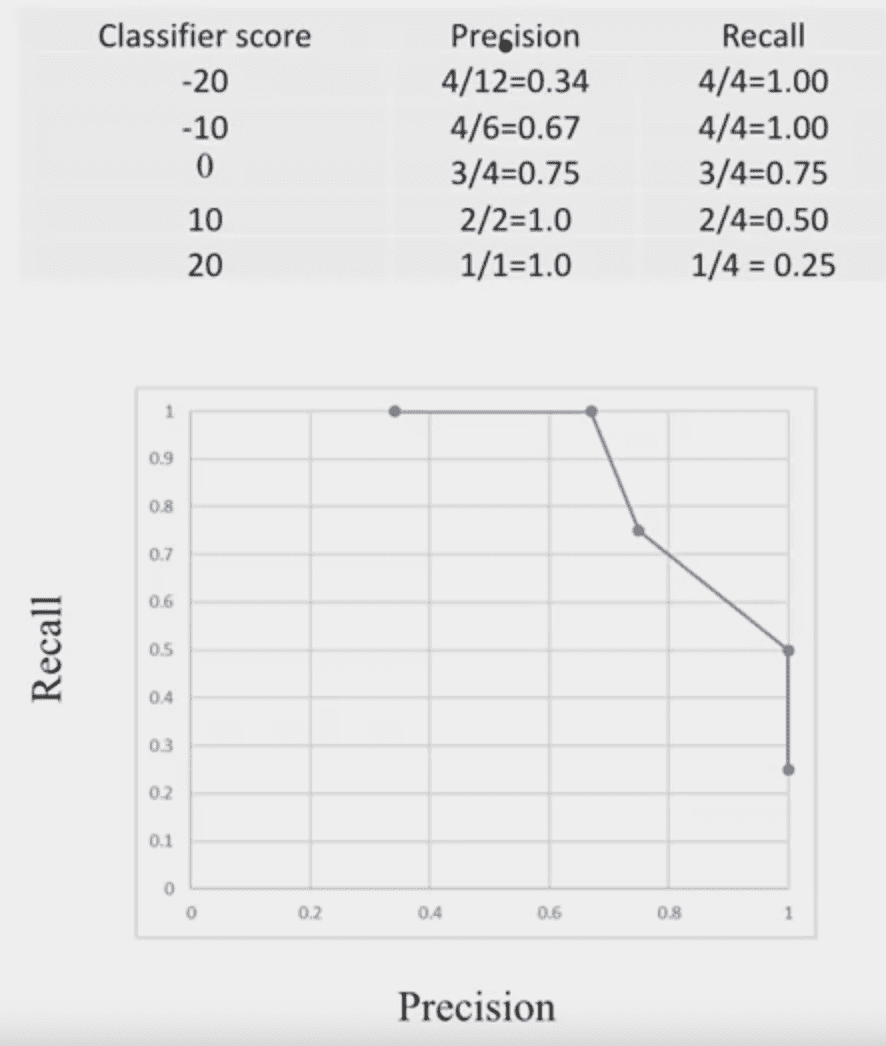# Precision Recall Curves

Right up corner is the ideal point:

• Recall = 1
• Precision = 1

“Speepness” of P-R curves is important:

• Maximize precision
• while maximizing recall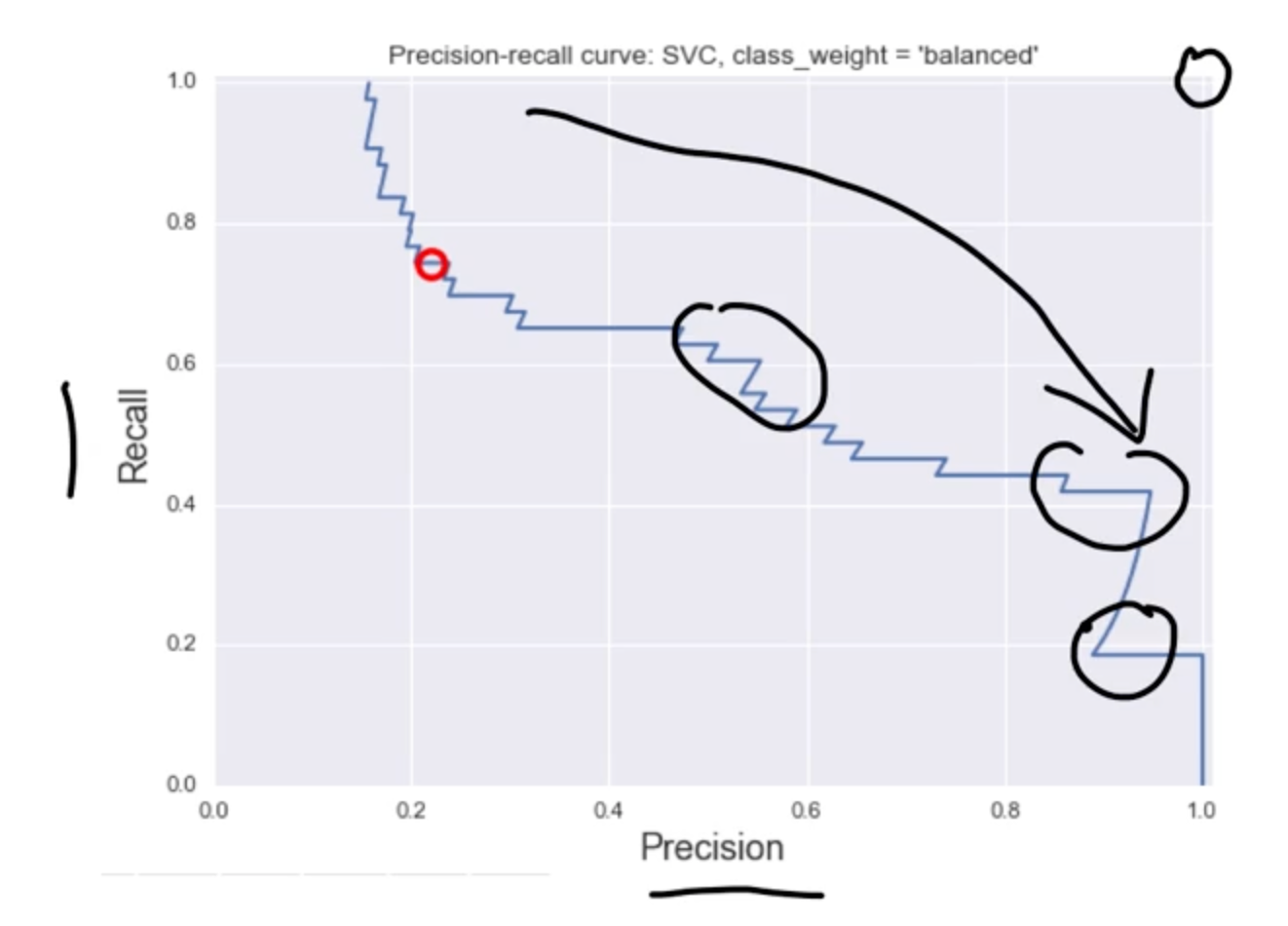from sklearn.metrics import precision_recall_curve

precision, recall, thresholds = precision_recall_curve(y_test, y_scores_lr)
closest_zero = np.argmin(np.abs(thresholds))
closest_zero_p = precision[closest_zero]
closest_zero_r = recall[closest_zero]

plt.figure()
plt.xlim([0.0, 1.01])
plt.ylim([0.0, 1.01])
plt.plot(precision, recall, label='Precision-Recall Curve')
plt.plot(closest_zero_p, closest_zero_r, 'o', markersize = 12, fillstyle = 'none', c='r', mew=3)
plt.xlabel('Precision', fontsize=16)
plt.ylabel('Recall', fontsize=16)
plt.axes().set_aspect('equal')
plt.show()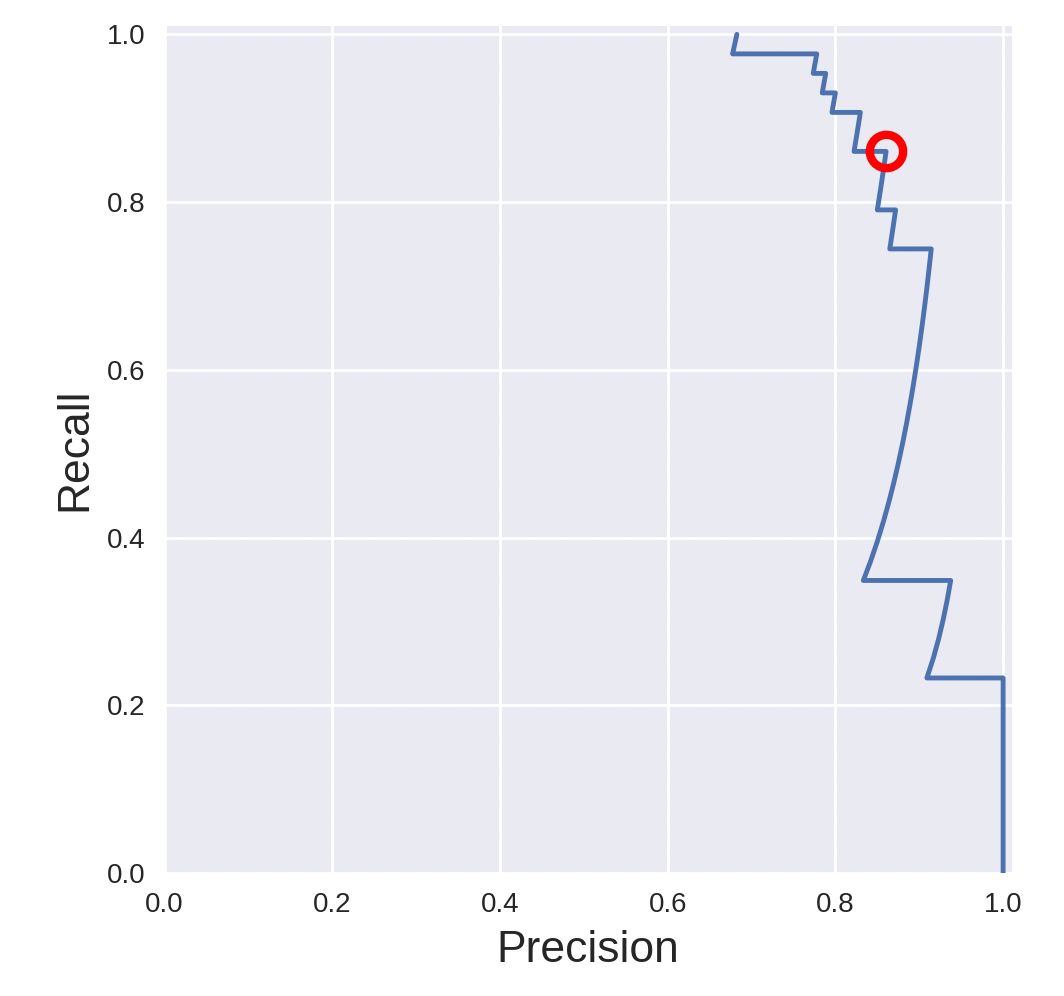## ROC Curves

ROC curves: receiver operating characteristic curves

The left up corner is the ideal point:

• False positive rate = 0
• True positive rate = 1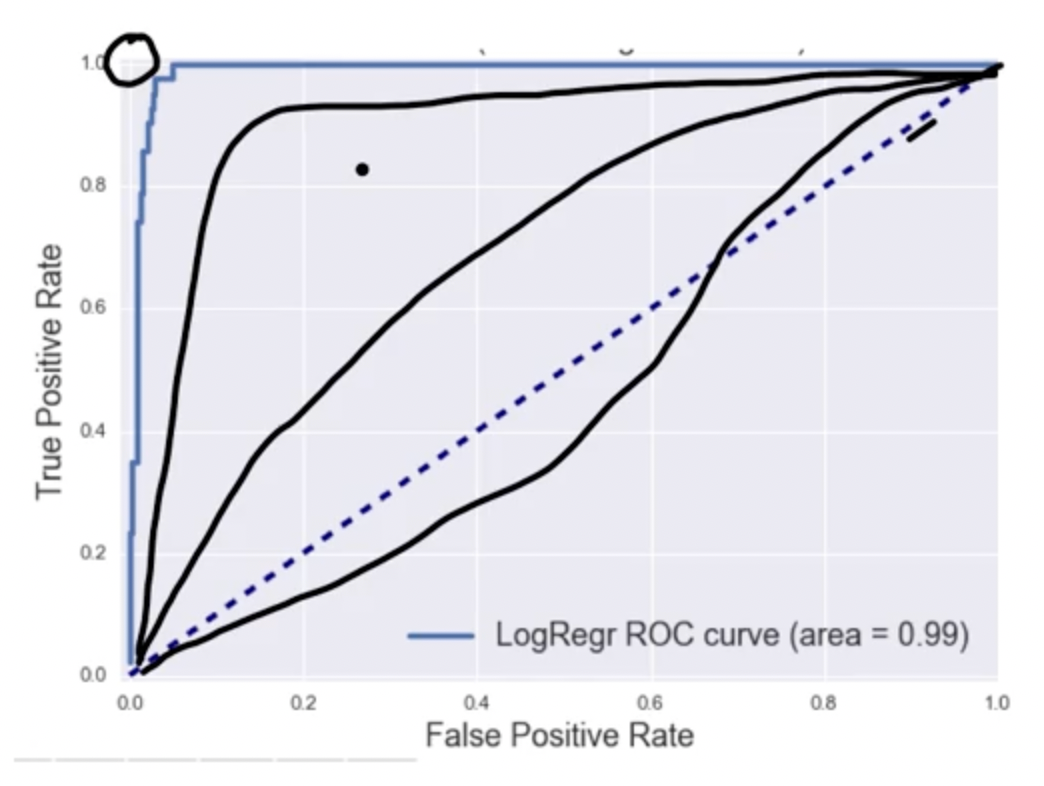## AUC

AUC stands for “Area Under the ROC Curve.” That is, AUC measures the entire two-dimensional area underneath the entire ROC curve (think integral calculus) from (0,0) to (1,1).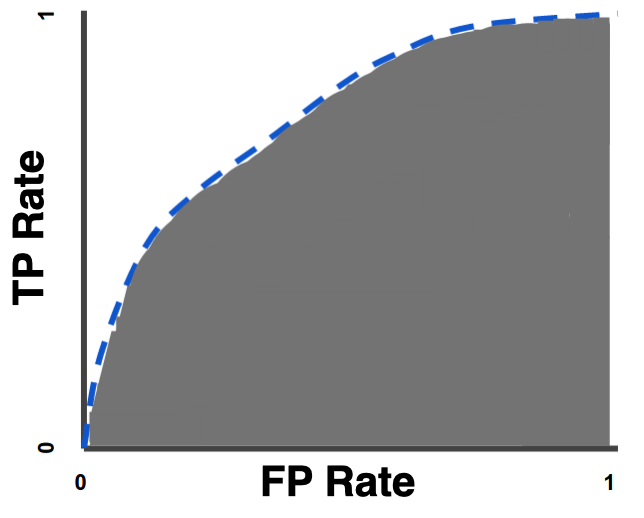## Application

from matplotlib import cm

X_train, X_test, y_train, y_test = train_test_split(X, y_binary_imbalanced, random_state=0)

plt.figure()
plt.xlim([-0.01, 1.00])
plt.ylim([-0.01, 1.01])
for g in [0.01, 0.1, 0.20, 1]:
svm = SVC(gamma=g).fit(X_train, y_train)
y_score_svm = svm.decision_function(X_test)
fpr_svm, tpr_svm, _ = roc_curve(y_test, y_score_svm)
roc_auc_svm = auc(fpr_svm, tpr_svm)
accuracy_svm = svm.score(X_test, y_test)
print("gamma = {:.2f}  accuracy = {:.2f}   AUC = {:.2f}".format(g, accuracy_svm,
roc_auc_svm))
plt.plot(fpr_svm, tpr_svm, lw=3, alpha=0.7,
label='SVM (gamma = {:0.2f}, area = {:0.2f})'.format(g, roc_auc_svm))

plt.xlabel('False Positive Rate', fontsize=16)
plt.ylabel('True Positive Rate (Recall)', fontsize=16)
plt.plot([0, 1], [0, 1], color='k', lw=0.5, linestyle='--')
plt.legend(loc="lower right", fontsize=11)
plt.title('ROC curve: (1-of-10 digits classifier)', fontsize=16)
plt.axes().set_aspect('equal')

plt.show()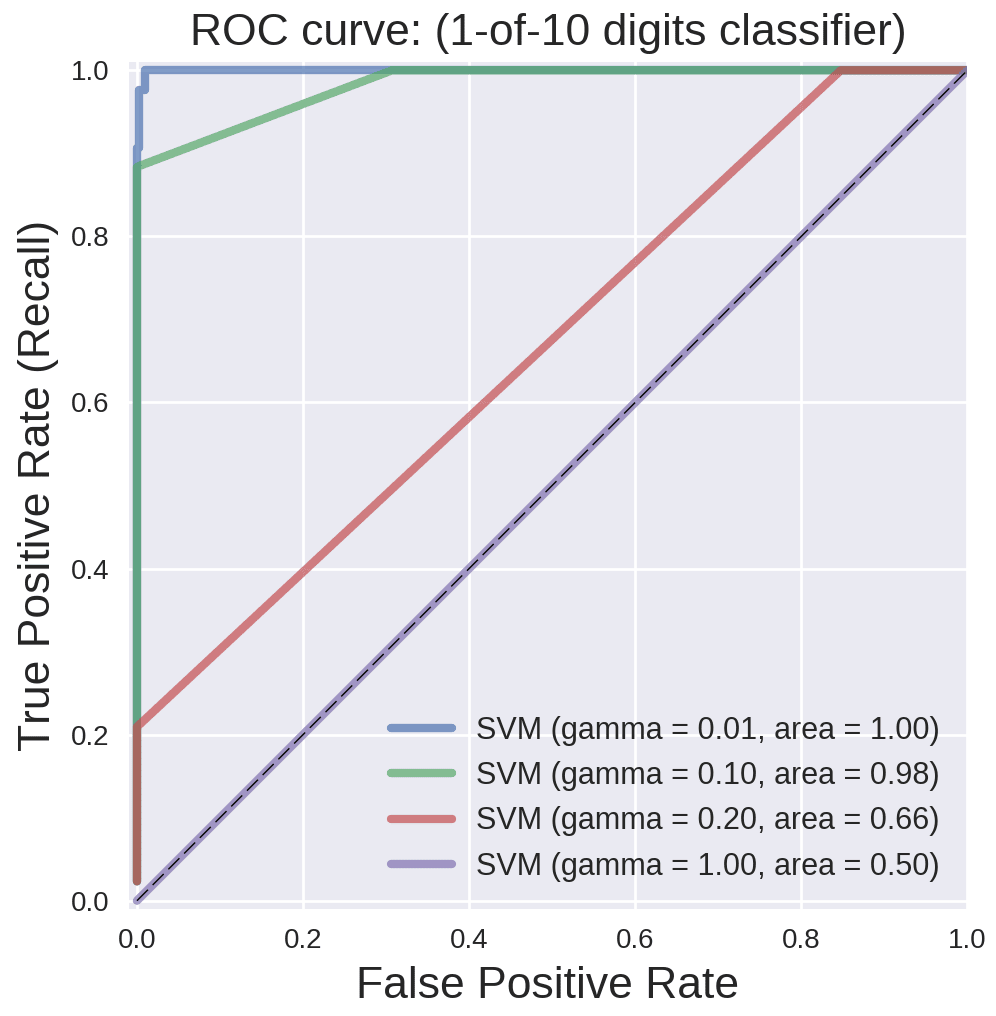# Multi-Class Evaluation

• A extension of the binary case
• Overall evaluation metrics are averages across classes
• Multi-label classification: each instance can have multiple labels

confusion matrix: can give clues

dataset = load_digits()
X, y = dataset.data, dataset.target
X_train_mc, X_test_mc, y_train_mc, y_test_mc = train_test_split(X, y, random_state=0)

svm = SVC(kernel = 'linear').fit(X_train_mc, y_train_mc)
svm_predicted_mc = svm.predict(X_test_mc)
confusion_mc = confusion_matrix(y_test_mc, svm_predicted_mc)
df_cm = pd.DataFrame(confusion_mc,
index = [i for i in range(0,10)], columns = [i for i in range(0,10)])

plt.figure(figsize=(5.5,4))
sns.heatmap(df_cm, annot=True)
plt.title('SVM Linear Kernel \nAccuracy:{0:.3f}'.format(accuracy_score(y_test_mc,
svm_predicted_mc)))
plt.ylabel('True label')
plt.xlabel('Predicted label')

svm = SVC(kernel = 'rbf').fit(X_train_mc, y_train_mc)
svm_predicted_mc = svm.predict(X_test_mc)
confusion_mc = confusion_matrix(y_test_mc, svm_predicted_mc)
df_cm = pd.DataFrame(confusion_mc, index = [i for i in range(0,10)],
columns = [i for i in range(0,10)])

plt.figure(figsize = (5.5,4))
sns.heatmap(df_cm, annot=True)
plt.title('SVM RBF Kernel \nAccuracy:{0:.3f}'.format(accuracy_score(y_test_mc,
svm_predicted_mc)))
plt.ylabel('True label')
plt.xlabel('Predicted label');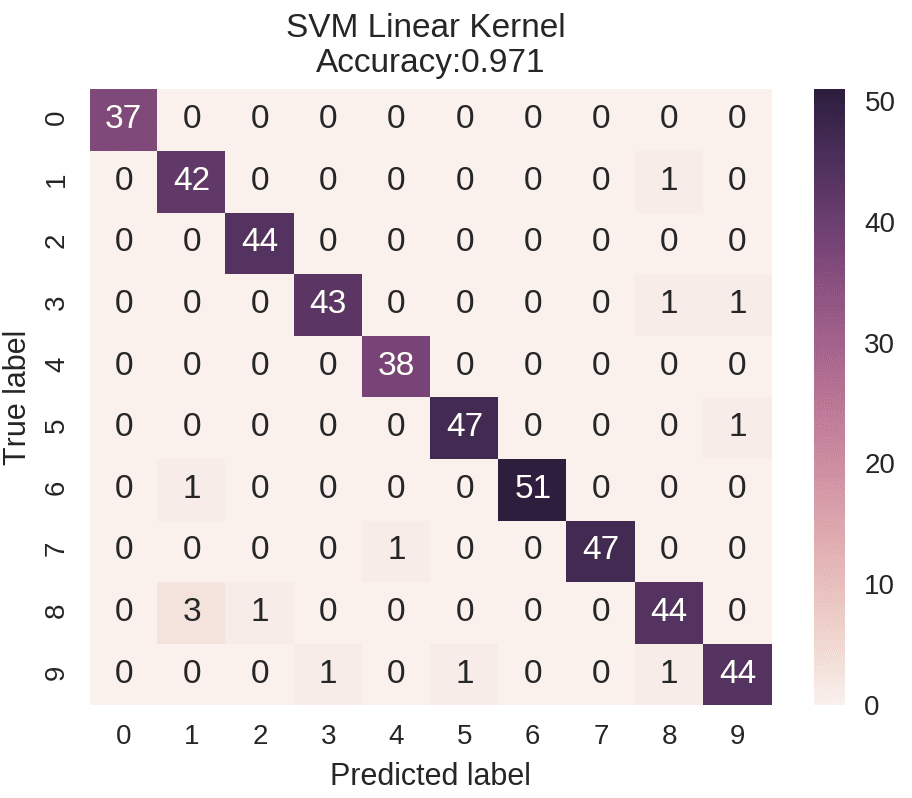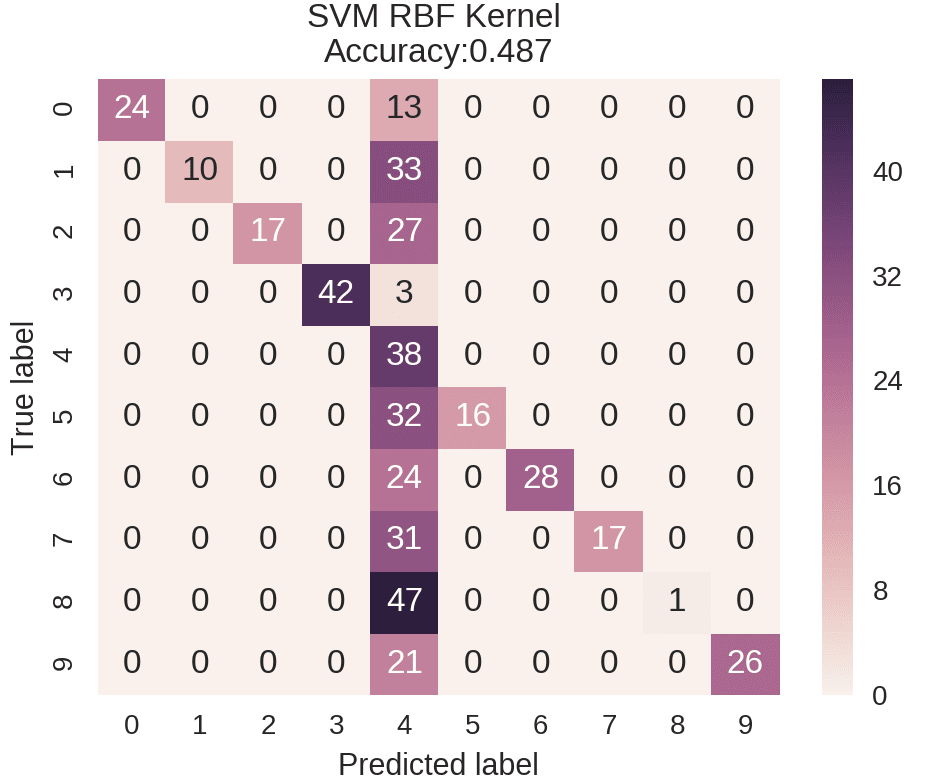## Macro-average precision

Each class have equal weight)

orange:0.2

lemon:0.5

apple:1.00

Macro-average precision = (0.2+0.5+1)/3 = 0.57

## Micro Average precision

Each instance has equal weight

Micro-average precision = precision:4/9 = 0.44

## How to choose?

• If some classes have more instances than others:
• Aim to weight your metric toward the largest ones, use micro-averaging.
• Aim to weight your metric toward the smallest ones, use macro-averaging.
• If the micro-average is much lower than the macro-average then examine the larger classes for poor metric performance
• If the macro-average is much lower than the micro-average then examine the smaller classes for poor metric performance

# Regression Evaluation

r2_score(best score = 1)

mean_absolute_error

mean_squard_error

median_absolute_error

Dummy regressor

%matplotlib notebook
import matplotlib.pyplot as plt
import numpy as np
from sklearn.model_selection import train_test_split
from sklearn import datasets
from sklearn.linear_model import LinearRegression
from sklearn.metrics import mean_squared_error, r2_score
from sklearn.dummy import DummyRegressor

X = diabetes.data[:, None, 6]
y = diabetes.target

X_train, X_test, y_train, y_test = train_test_split(X, y, random_state=0)

lm = LinearRegression().fit(X_train, y_train)
lm_dummy_mean = DummyRegressor(strategy = 'mean').fit(X_train, y_train)

y_predict = lm.predict(X_test)
y_predict_dummy_mean = lm_dummy_mean.predict(X_test)

print('Linear model, coefficients: ', lm.coef_)
print("Mean squared error (dummy): {:.2f}".format(mean_squared_error(y_test,
y_predict_dummy_mean)))
print("Mean squared error (linear model): {:.2f}".format(mean_squared_error(y_test, y_predict)))
print("r2_score (dummy): {:.2f}".format(r2_score(y_test, y_predict_dummy_mean)))
print("r2_score (linear model): {:.2f}".format(r2_score(y_test, y_predict)))

# Plot outputs
plt.scatter(X_test, y_test,  color='black')
plt.plot(X_test, y_predict, color='green', linewidth=2)
plt.plot(X_test, y_predict_dummy_mean, color='red', linestyle = 'dashed',
linewidth=2, label = 'dummy')

plt.show()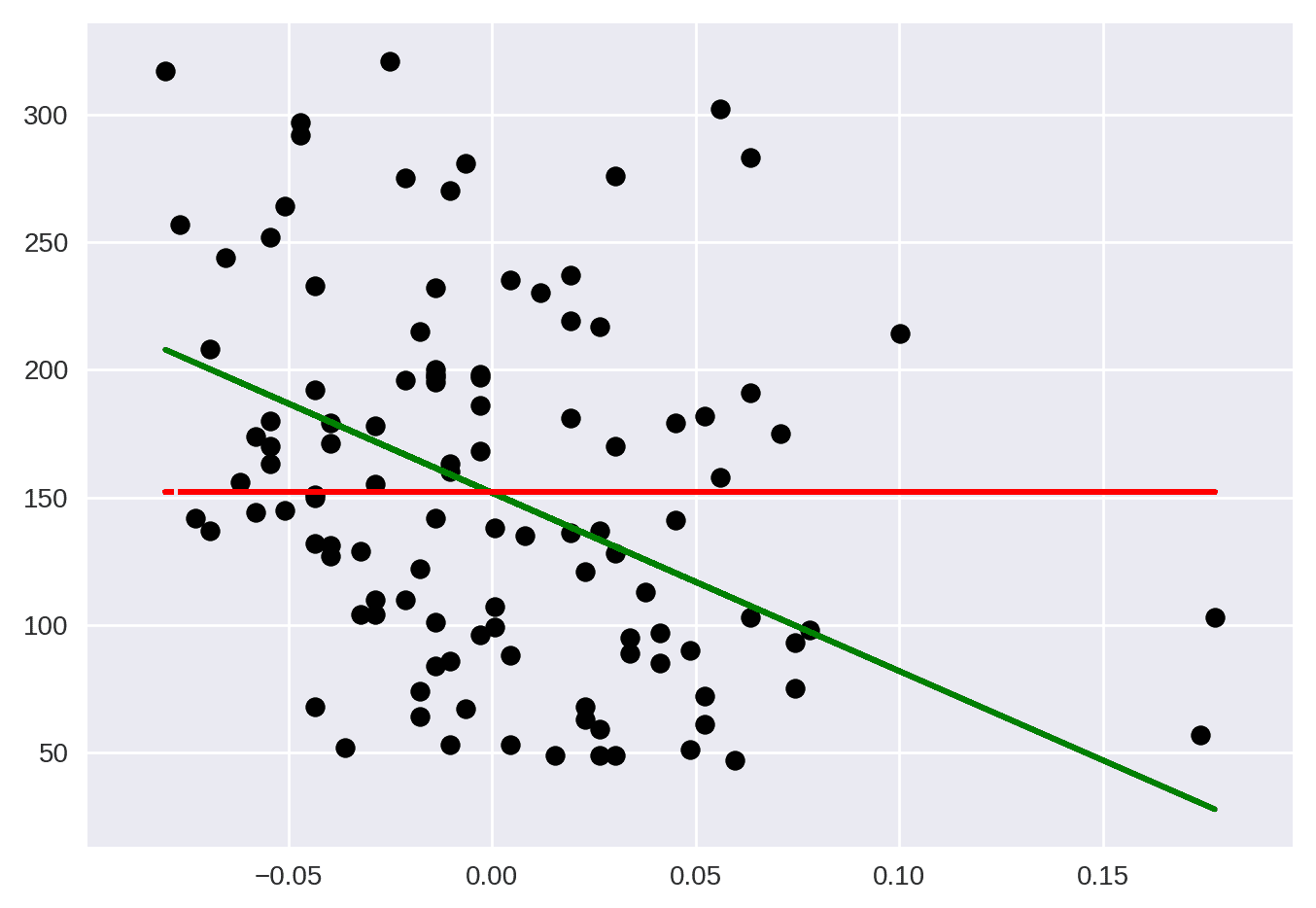# Model Selection

## Training Validation and Test Framework

Use 3 data splits:

1. Training set(model building)
2. Validation set(model selection)
3. Test set(final evaluation)

In practice:

1. Create an initial training /test split
2. Do cross-validation on the training data for model/parameter selection
3. Save the held-out test set for final model evaluation

## Application

### Cross-validation example

from sklearn.model_selection import cross_val_score
from sklearn.svm import SVC

# again, making this a binary problem with 'digit 1' as positive class
# and 'not 1' as negative class
X, y = dataset.data, dataset.target == 1
clf = SVC(kernel='linear', C=1)

# accuracy is the default scoring metric
print('Cross-validation (accuracy)', cross_val_score(clf, X, y, cv=5))
# use AUC as scoring metric
print('Cross-validation (AUC)', cross_val_score(clf, X, y, cv=5, scoring = 'roc_auc'))
# use recall as scoring metric
print('Cross-validation (recall)', cross_val_score(clf, X, y, cv=5, scoring = 'recall'))


Cross-validation (accuracy) [ 0.91944444 0.98611111 0.97214485 0.97493036 0.96935933]
Cross-validation (AUC) [ 0.9641871 0.9976571 0.99372205 0.99699002 0.98675611]
Cross-validation (recall) [ 0.81081081 0.89189189 0.83333333 0.83333333 0.83333333]

### Grid Search example

from sklearn.svm import SVC
from sklearn.model_selection import GridSearchCV
from sklearn.metrics import roc_auc_score

X, y = dataset.data, dataset.target == 1
X_train, X_test, y_train, y_test = train_test_split(X, y, random_state=0)

clf = SVC(kernel='rbf')
grid_values = {'gamma': [0.001, 0.01, 0.05, 0.1, 1, 10, 100]}

# default metric to optimize over grid parameters: accuracy
grid_clf_acc = GridSearchCV(clf, param_grid = grid_values)
grid_clf_acc.fit(X_train, y_train)
y_decision_fn_scores_acc = grid_clf_acc.decision_function(X_test)

print('Grid best parameter (max. accuracy): ', grid_clf_acc.best_params_)
print('Grid best score (accuracy): ', grid_clf_acc.best_score_)

# alternative metric to optimize over grid parameters: AUC
grid_clf_auc = GridSearchCV(clf, param_grid = grid_values, scoring = 'roc_auc')
grid_clf_auc.fit(X_train, y_train)
y_decision_fn_scores_auc = grid_clf_auc.decision_function(X_test)

print('Test set AUC: ', roc_auc_score(y_test, y_decision_fn_scores_auc))
print('Grid best parameter (max. AUC): ', grid_clf_auc.best_params_)
print('Grid best score (AUC): ', grid_clf_auc.best_score_)


• 534
• 0

### YOU MIGHT ALSO LIKE

0 0 vote
Article Rating
Subscribe0 评论
Inline Feedbacks